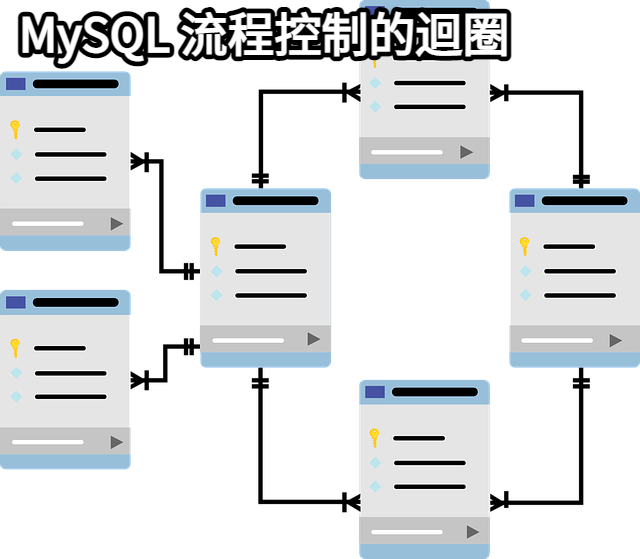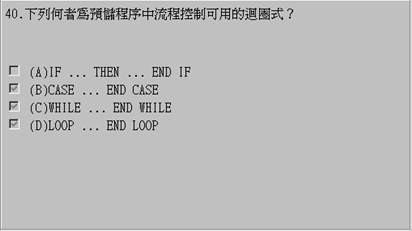# MySQL 流程控制的迴圈1. REPEAT
2. WHILE
3. LOOP, LEAVE & ITERATE

IF, CASE, LOOP, WHILE, ITERATE, 及LEAVE 構造被完全實現。

#### 20.2.12.1. IF 語句

`IF search_condition THEN statement_list`
`    [ELSEIF search_condition THEN statement_list ] ...`
`    [ELSE statement_list ]`
`END IF`

IF 實現了一個基本的條件構造。如果search_condition求值為真，相應的SQL語句列表被執行。如果沒有search_condition匹配，在ELSE子句裡的語句列表被執行。statement_list可以包括一個或多個語句。

#### 20.2.12.2. CASE語句

`CASE case_value`
`    WHEN when_value THEN statement_list`
`    [WHEN when_value THEN statement_list ] ...`
`    [ELSE statement_list ]`
`END CASE`

Or:

`CASE`
`    WHEN search_condition THEN statement_list`
`    [WHEN search_condition THEN statement_list ] ...`
`    [ELSE statement_list ]`
`END CASE`

#### 20.2.12.3. LOOP語句

`[ begin_label :] LOOP`
`    statement_list`
`END LOOP [ end_label ]`

LOOP 允許某特定語句或語句群的重複執行，實現一個簡單的迴圈構造。在迴圈內的語句一直重複直到迴圈被退出，退出通常伴隨著一個LEAVE語句。

LOOP語句可以被標註。除非begin_label存在，否則end_label不能被給出，並且如果兩者都出現，它們必須是同樣的。

#### 20.2.12.4. LEAVE語句

`LEAVE label`

#### 20.2.12.5. ITERATE語句

`ITERATE label`

ITERATE只可以出現在LOOP, REPEAT,和WHILE語句內。ITERATE意思為：“再次迴圈。”

`CREATE PROCEDURE doiterate(p1 INT)`
`BEGIN`
`  label1: LOOP`
`    SET p1 = p1 + 1;`
`    IF p1 < 10 THEN ITERATE label1; END IF;`
`    LEAVE label1;`
`  END LOOP label1;`
`  SET @x = p1;`
`END`

#### 20.2.12.6. REPEAT語句

`[ begin_label :] REPEAT`
`    statement_list`
`UNTIL search_condition`
`END REPEAT [ end_label ]`

REPEAT語句內的語句或語句群被重複，直至search_condition 為真。

REPEAT語句可以被標註。除非begin_label也存在，end_label才能被用，如果兩者都存在，它們必須是一樣的。

`mysql> delimiter //`
`mysql> CREATE PROCEDURE dorepeat(p1 INT)`
`    -> BEGIN`
`    -> SET @x = 0;   `
`    -> REPEAT SET @x = @x + 1; UNTIL @x > p1 END REPEAT;   `
`    -> END`
`    -> //`
`Query OK, 0 rows affected (0.00 sec)`
`mysql> CALL dorepeat(1000)//`
`Query OK, 0 rows affected (0.00 sec)`
`mysql> SELECT @x//`
`+------+`
`| @x    |`
`+------+`
`| 1001 |`
`+------+`
`1 row in set (0.00 sec)`

#### 20.2.12.7. WHILE語句

`[ begin_label :] WHILE search_condition DO`
`    statement_list`
`END WHILE [ end_label ]`

WHILE語句內的語句或語句群被重複，直至search_condition 為真。

WHILE語句可以被標註。除非begin_label也存在，end_label才能被用，如果兩者都存在，它們必須是一樣的。

`CREATE PROCEDURE dowhile()`
`BEGIN`
`  DECLARE v1 INT DEFAULT 5;`
`  WHILE v1 > 0 DO`
`    ...`
`    SET v1 = v1 - 1;`
`  END WHILE;`
`END`

## mysql for loop

```drop procedure if exists sum1;
create procedure sum1(a int)
begin
declare sum int default 0;
declare i int default 1;

while i <= a DO -- 迴圈開始
set sum = sum + i;
set i = i + 1;
end while; -- 迴圈结束

select sum; -- 印出結果
end
-- 執行
call sum1(10);```